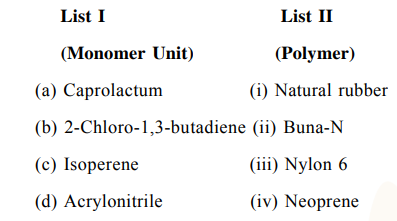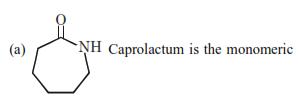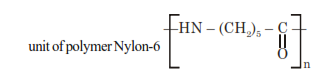# Match List I with List II.`
Question:

Match List I with List II.Choose the correct answer from the options given below :

1. (a) $\rightarrow$ (iv), (b) $\rightarrow$ (iii), (c) $\rightarrow$ (ii), (d) $\rightarrow$ (i)

2. (a) $\rightarrow$ (ii), (b) $\rightarrow$ (i), (c) $\rightarrow$ (iv), (d) $\rightarrow$ (iii)

3. (a) $\rightarrow$ (iii), (b) $\rightarrow$ (iv), (c) $\rightarrow$ (i), (d) $\rightarrow$ (ii)

4. (a) $\rightarrow$ (i), (b) $\rightarrow$ (ii), (c) $\rightarrow$ (iii), (d) $\rightarrow$ (iv)

Correct Option: , 3

Solution:(b) 2-Chlorobuta- 1,3 -diene is the monomeric unit of polymer neoprene.

(c) 2-Methylbuta-1, 3-diene is the monomeric unit of polymer natural rubber.

(d) $\mathrm{CH}_{2}=\mathrm{CH}-\mathrm{CN}$ (Acrylonitrile) is the one of the monomeric unit of polymer Buna-N